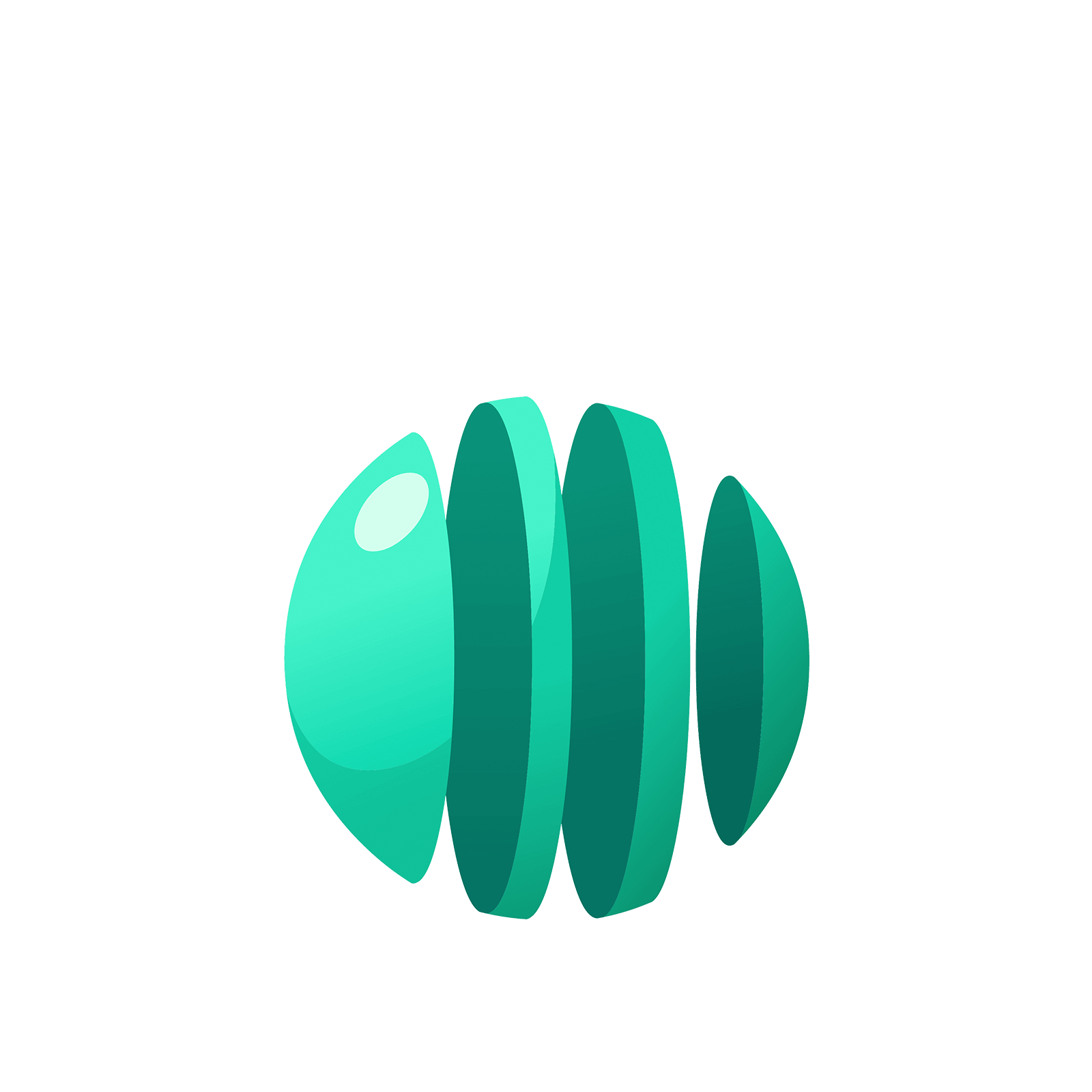06 285 000# Station  8FDecimals in Other BasesWho said we need to stay with a $1 \leftarrow 10$ machine?

### Question 1

The following picture shows that $1432 \div 13 = 110 R 2$ in a $1 \leftarrow 5$ machine.If we work with reciprocals of powers of five we can keep unexploding dots and continue the division process.Here goes!(This reads as $1432 \div 13 = 110.1 R 0.2$ if you like.)(This reads as $1432 \div 13 = 110.11 R 0.02$ if you like.)And so on.
We get, as a statement of base $5$ arithmetic,

$1432 \div 13 = 110.1111...$.

To translate this to ordinary arithmetic we have that

$1432$ in base five is $1 \times 125 + 4 \times 25 + 3 \times 5 + 1 \times 1 = 242$,

$13$ in base five is $1 \times 5 + 3 \times 1 = 8$,

$110.111...$ in base five is $30 + \dfrac{1}{5} + \dfrac{1}{25} + \dfrac{1}{125} + ...$,

so we are claiming, in ordinary arithmetic, that

$242 \div 8 = 30 + \dfrac{1}{5} + \dfrac{1}{25} + \dfrac{1}{125} + ...$.

Whoa!

What does the geometric series formula from Infinitia say about the sum $\dfrac{1}{5} + \dfrac{1}{25} + \dfrac{1}{125} + ...$. Does it equal a quarter?

To be clear, on Infinitia we showed that

$1+x+x2+x3+...=\dfrac{1}{1-x}$.

Multiplying through by $x$ then gives $x+x2+x3+...=\dfrac{x}{1-x}$.

Perhaps it is this second version of the geometric formula we need to work with now.)

Here are some (challenging) practice questions if you are up for them.

Compute $8 \div 3$ in a base $10$ machine and show that it yields the answer $2.666...$.

### Question 2

Compute $1 \div 11$ as a problem in base $3$ and show that it yields the answer $0.0202020202...$.
(In base three, “$11$” is the number four, and so this question establishes that the fraction $\dfrac{1}{4}$ written in base three is $0.0202020202...$.)

### Question 3

Show that the fraction $\dfrac{2}{5}$, written here in base ten, has the “decimal” representation $0.121212...$ in base four. (That is, compute $2 \div 5$ in a $1 \leftarrow 4$ machine.)

### Question 4

CHALLENGE

What fraction has decimal expansion $0.3333...$ in base $7$? Is it possible to answer this question by calling this number $x$ and multiplying both sides by $10$? (Does “$10$” represent ten?)

### Question 5

Use an $1 \leftarrow x$ machine and $x$-mals to show that $\dfrac {1}{x-1} = \dfrac{1}{x} + \dfrac{1}{x^2} + \dfrac{1}{x^3} + \dfrac{1}{x^4} + ...$.You can either play with some of the optional stations below or go to the next island!